• ## 指针与一维数组

千次阅读 2018-07-28 15:15:12
对于数组a，a的值&amp;a相等 int a[] = { 1,2,3,4,5 }; int *p = a; printf("%p %p %p\n", a, &amp;a, p); 我们发现三者地址相同 2.指针操作数组 1）把指针当作数组名来使用 ...
1.数组名
数组名本身就是一个指针（地址），它代表了数组的首地址，数组在内存中连续存放
对于一个数组a，a的值与&a相等
int a[] = { 1,2,3,4,5 };
int *p = a;

printf("%p  %p  %p\n", a, &a, p);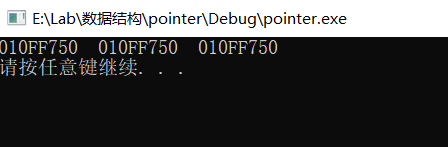我们发现三者地址相同
2.指针操作数组
1）把指针当作数组名来使用
格式：指针名[偏移位置]
int a[] = { 1,2,3,4,5 };
int *p = a;

printf("%p\n", p);
for (int i = 0; i < 5; i++)
{
printf("%d  ", p[i]);
}
printf("\n");
printf("%p\n", p);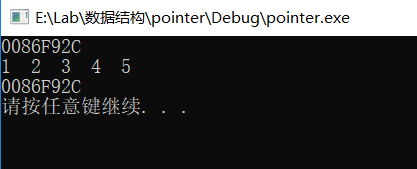2）指针偏移
格式：*(指针名+偏移位置)
指针+1或-1是向上或向下偏移sizeof(int)个字节
int a[] = { 1,2,3,4,5 };
int *p = a;

printf("%p\n", p);
for (int i = 0; i < 5; i++)
{
printf("%d  ", *(p+i));
}
printf("\n");
printf("%p\n", p);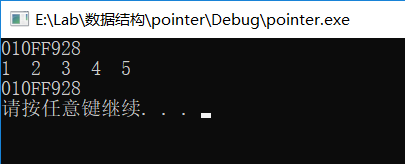3）指针自身++来获取每个元素的位置
格式：*(指针名++)
指针++或--是向上或向下移动sizeof(int)个字节
int a[] = { 1,2,3,4,5 };
int *p = a;

printf("%p\n", p);
for (int i = 0; i < 5; i++)
{
printf("%d  ", *(p++));
}
printf("\n");
printf("%p\n", p);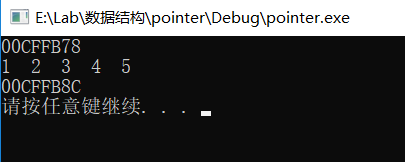从上面三种操作方式上来看，我们可以发现，在第一种和第二种中，指针本身的位置并没有改变，在循环完后，指针还是指在数组的首地址，但是在第三种方式中，指针的位置改变了，偏移量正好是20个字节，表示在方法三中，指针是通过自己的移动来读取元素的位置的
3.数组自身的读取方式
1）数组名[偏移位置]
int a[] = { 1,2,3,4,5 };

printf("%p\n", a);
for (int i = 0; i < 5; i++)
{
printf("%d  ", a[i]);
}
printf("\n");
printf("%p\n", a);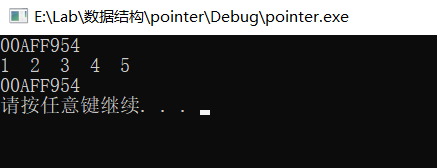2）*(数组名+偏移位置)
int a[] = { 1,2,3,4,5 };

printf("%p\n", a);
for (int i = 0; i < 5; i++)
{
printf("%d  ", *(a+i));
}
printf("\n");
printf("%p\n", a);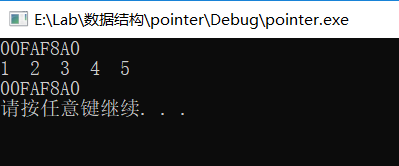3）模仿指针变量的自身++，*(数组名++)
int a[] = { 1,2,3,4,5 };

printf("%p\n", a);
for (int i = 0; i < 5; i++)
{
printf("%d  ", *(a++));
}
printf("\n");
printf("%p\n", a);
编译出错：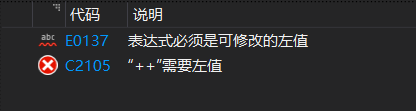从错误提示中就可以发现，数组名虽也是一个指针，指向数组元素的首地址，这与指针变量不同的是，数组名是一个常量，不能改变其值，也就自然不能用于++和--了，所以出现错误
4.数组名与指针的区别
类型是否可变可运算数组名不可变，是常量无法++和--指针可以变，是变量可以++和--
5.指针型数组
用于存放地址的指针数组，每个元素都是地址值
格式：指针类型   数组名[N]
int *a;      //整型指针数组
char *b;     //字符型指针数组
float *c;    //浮点型针数组
int a = 10;
int b = { 1,2,3 };

int *p = { &a,b,&b };

for (int i = 0; i < 3; i++)
{
printf("%p  ", *(p+i));
}
printf("\n");

for (int i = 0; i < 3; i++)
{
printf("%d  ", *(*(p + i)));
}
printf("\n");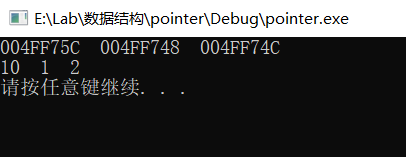代码中，p是一个指针型数组，所以它也是一个数组名，满足数组名的特性。即它是一个常量，不可实现++和--运算，它里面存放的是地址，用*(p+i)是访问它的第i个元素，该元素是地址；用*(*(p+i))是访问它第i个元素地址中存放的值，也说明p是一个指针的指针
展开全文• 1、 对于任意二维数组 int a[ROW][COLUMN] 来说， a+i 表示行指针，而 a[i] 标识列指针（其中 i 表示某个整形值） ，行指针转化为列指针是通过在行指针前面加“ * ”运算符做到，而列指针转化为行指针是通过“ &...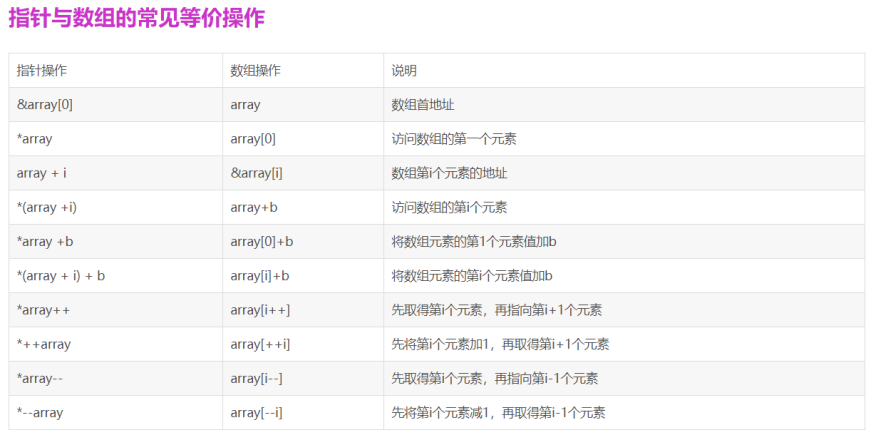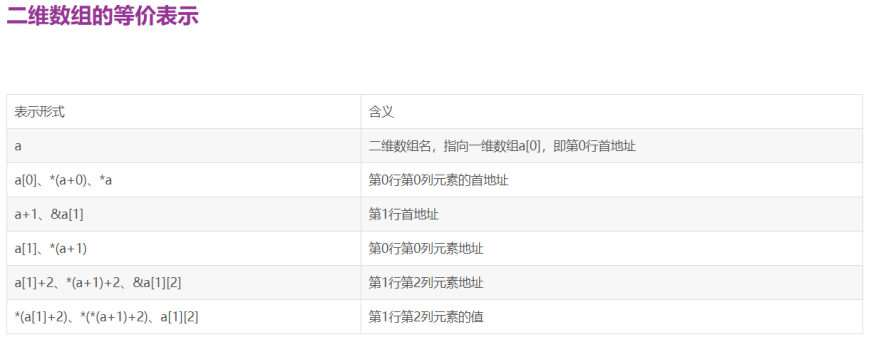1、 对于任意二维数组 int a[ROW][COLUMN] 来说， a+i 表示行指针，而 a[i] 标识列指针（其中 i 表示某个整形值） ，行指针转化为列指针是通过在行指针前面加“ * ”运算符做到，而列指针转化为行指针是通过“ & ”来做到。如 Fig.1 所示：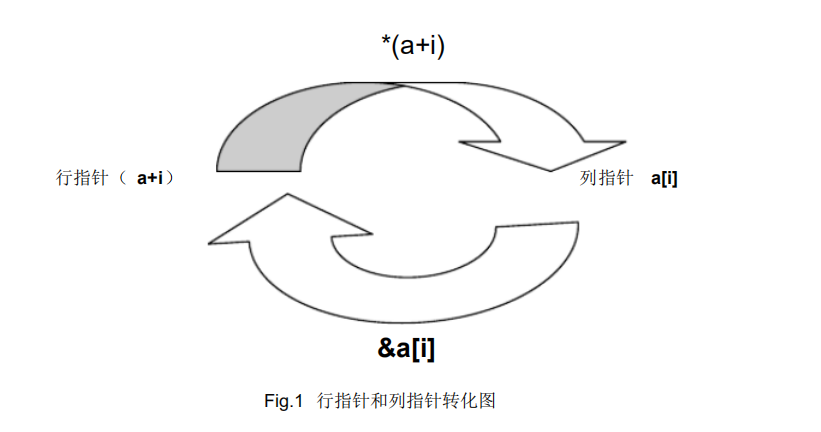2、 在二维数组 int a[ROW][COLUMN] 中，可以认为划分为三层，最高层为行指针，中间层为列指针，而最底层为元素。由高层到底层可以通过 ”*”运算符来做到，而由底层到高层可以通过 ”& ”运算符来做到。如 Fig.2 所示：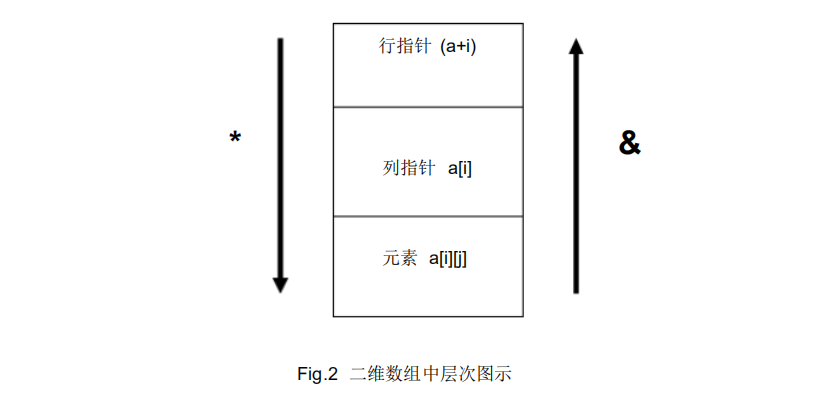3、 对于二维数组 int a[ROW][COLUMN] 来说，行指针的本质就是一个指向包含 COLUMN个元素一维数组的指针 也就是 int (*p)[COLUMN] ，而列指针的本质就是一个指向元素的指针，也就是 int *p;
4、 对于一维数组来说，总结出的一个通用公式是 a[x]= * (a+x) = * (x+a)=x[a] ，这也就是我们既可以用 a也可以用 3[a]的原因；而对于二维数组来说，总结出的通用公式是a[i][j]=* ( *(a+i) +j )
展开全文• 指针一维数组的讲解，本人觉得挺好的，给大家分享下，适合一些小白c++基础
• 指针与一维数组： #include  void test1() {  //利用指针来修改数组元素  int a;  int *p;  p = &a; // p = a; 等效  *p = 10;  printf("%d\n",a); } void test2() {  /...
指针与一维数组：
#include <stdio.h>
void test1()
{
//利用指针来修改数组元素
int a;
int *p;
p = &a; //    p = a; 等效
*p = 10;
printf("%d\n",a);
}
void test2()
{
//遍历数组元素
int a = {1,2,3};
for (int i=0; i<3; i++)
{
printf("a[%d]的值为%d\n",i,a[i]);
}
}
void test3()
{
//利用数组来遍历数组元素
int a={1,2,3};
int *p;
p = a;

for (int i=0; i<3; i++)
{
//注意：我们这里的p+1是加的对应数据类型的一个单元
//遍历完，p的值没有改变
printf("a[%d]的值为%d\n",i,*(p+i));
}
}
void test4()
{
//利用数组来遍历数组元素
int a={1,2,3};
int *p = a;
for (int i=0; i<3; i++)
{
//注意：我们这里的p+1是加的对应数据类型的一个单元
//遍历完，p的值改变了，指向了a p=p+1
printf("a[%d]的值为%d\n",i,*(p++));
}
}
void test5()
{
//利用数组来遍历数组元素
int a={1,2,3};
int *p;
p = a;
for (int i=0; i<3; i++)
{
//注意：我们这里的p+1是加的对应数据类型的一个单元
//a为数组的首地址，用a+i也行， 但是a++不行，因为a是常量，数组的首地址是不能修改的
printf("a[%d]的值为%d\n",i,*(a+i));
}
}
void test6()
{
//利用数组来遍历数组元素
int a={1,2,3};
int *p = a;
for (int i=0; i<3; i++,p++)
{
//注意：我们这里的p+1是加的对应数据类型的一个单元
printf("a[%d]的值为%d\n",i,*p);
}
}
void test7()
{
//利用数组来遍历数组元素
int a={1,2,3};
int *p = a;
for (int i=0; p<a+3; i++,p++)
{
//注意：我们这里的p+1是加的对应数据类型的一个单元
printf("a[%d]的值为%d\n",i,*p);
}
}

int main(int argc, const char * argv[])
{
test1();
test2();
test3();
test4();
test5();
test6();
test7();
return 0;
}
展开全文• 但在内存中，a 的分布是一维线性的，整个数组占用一块连续的内存： C语言中的二维数组是按行排列的，也就是先存放 a 行，再存放 a 行，最后存放 a 行；每行中的 4 个元素也是依次存放。数组 a
• 指针与一维数组 指针移动方式访问数组元素： int a, *p; // a存放了数组的首地址 p=a; // 数组首地址赋给p p++; // 向下移动4个字节 指针与数组名都是地址，因此可以混合使用访问数组元素。...

C编译程序用数组名存放数组在内存中的首地址。
指针访问内存比变量名访问速度更快，数组采用指针加减1的方式访问数组，增加了访问内存的灵活性。

指针与一维数组
指针移动方式访问数组元素：
int a, *p;     // a存放了数组的首地址
p=a;     // 数组首地址赋给p
p++;     // 向下移动4个字节
指针与数组名都是地址，因此可以混合使用访问数组元素。
int *pa=a; 和 int *pa=&a;（// a的首地址）两者完全等价！
pa和a可以混合使用的图片说明，前面一列是地址（a和pa都表示地址），加*表示里面的元素。指针还可以移动，如图2。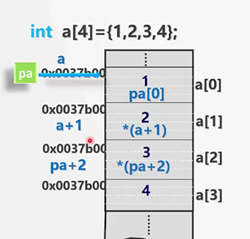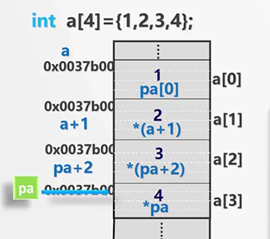数组元素的等价引用形式：
a[i]
pa[i]   // pa和a可以混合使用，pa和a都表示首地址
*(a+i)   // 表示地址的前面加一个*，就代表该地址里面的元素
*(pa+i)   // pa和a混合使用
对应的数组元素的地址表现形式：
&a[i]    // 加取地址符号就表示地址
&pa[i]
(a+i)    // *和&互为逆运算，因此前面没有了符号
(pa+i)
注意（区别）：
数组名是指针常量，不是指针变量，因此不能给数组名赋值！但指针可以。例如：pa=pa+1这是可以的，但是不能有a=a+1这种操作，任何修改数组名的操作都是错误的！
程序1：用指针访问数组，计算数组的元素之和。
/* 用指针访问数组，计算数组的元素之和 */
#include<stdio.h>
#include<stdlib.h>
int main()
{
int iarray={0,2,4,6,8,10,12,14,16,18},*p=iarray;
int i,sum=0;
for(i=0;i<10;i++)
{
sum+=*p;
p++;        // sum+=*iarray; iarray++是错误的！数组名不能进行自增操作！
｝
printf("sum is %d\n",sum);
system("pause");
return 0;
}
注意：p++并不是简单的加1，而是加了它的基类型所占的字节数，即移动了sizeof(int)=4个字节。sizeof()宏定义可获取所占字节数。
指针快速访问数组，但也只能逐个处理，不能一次性处理所有元素。
用指针进行数组元素的输入输出（同样只能一次处理一个元素，用for循环）：
for(i=0;i<4;i++)
{
scanf_s(“%d”,&a[i]);   // 输入
printf(“%d”,a[i]);   // 输出
}
for(p=a;p<(a+4);p++)
{
scanf_s(“%d”,p);   // 用指针输入，p本身是个地址，不用再加取地址符号
printf(“%d”,*p);   // 用指针输出
}
访问数组元素的方法（五种，可混合使用）：
void main()    // 定义和初始化
{
int sum1,sum2,sum3,sum4,sum5;
int iarray[]={1,3,2,5,2,56,34,2,7,60};
int *iptr;
int size,n;

sum1=sum2=sum3=sum4=sum5=0;
size=sizeof(iarray)/sizeof(*iarray);
}
第一种：数组名和下标的方式
for(n=0;n<size;n++)
{
sum1+=iarray[n];
}
第二种：通过移动指针，逐个元素的访问
iptr=iarray;
for(n=0;n<size;n++)
{
sum2+=*iptr;
}
第三种：通过指针和间接地址的方式，访问数组的所有元素
iptr=iarray;
for(n=0;n<size;n++)
{
sum3+=*(iptr+n);
}
第四种：通过指针，引用下标的方式，按照数组的方式访问数组所有元素
iptr=iarray;
for(n=0;n<size;n++)
{
sum4+=iptr[n];
}
第五种：通过数组名加偏移量的方式
for(n=0;n<size;n++)
{
sum5+=*(iarray+n);
}
求数组的首地址（两种）：
第一种：通过数组名获得首地址（数组名）
第二种：通过数组的第一个元素来获得首地址（首个元素地址），这里指针变量p是通过数组名获得指向数组a的第一个元素。
通过数组首地址访问数组元素的方式：
第一种：直接访问（数组名+下标变量，如a）
第二种：指针加偏移量的间接地址访问，如*(p+i)
第三种：用数组名做地址值（指针值）的间接地址访问，如与a[i]等价的语句为*(a+i)
第四种：将指针变量看做数组名，再加下标变量，如p[i]。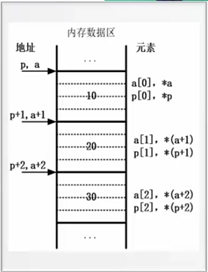总结：
数组名是表示数组元素的连续空间的首地址，可以看做一个“常量”指针，它的值不能被修改，即不能修改其指向。数组元素可通过 下标法来引用，也可通过指针来引用，还可以混合使用。同样地，指针也可以当做一个数组名来使用。（方便、灵活、易混淆、易出错）

指针与二维数组
C语言是按照行优先的原则存储数组的。
int a;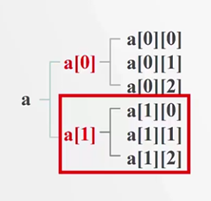这样理解：我们把每一行看成一个数据元素，那么就有a和a两个元素（两个一维数组），地址分别为a+0和a+1。
注意：这里a[i]不表示元素，还是表示地址，是这一行一维数组的首地址，那么a[i]+j就表示第i行第j个元素的地址。即a[i]+j和&a[i][j]完全等价（都表示第i行第j列元素的地址），a[i][j]和*a[i]+j完全等价（都表示第i行第j列的元素）。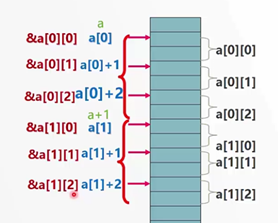二维数组与行：
(1) a代表二维数组的首地址，也是第0行的首地址；
(2) a+i代表第i行元素的首地址，即&a[i]；
(3) a+i或&a[i]表示行地址，每次加一表示会移动到下一行；
(4) *(a+i)即a[i]，不代表具体元素，依然是个地址（第i行的首地址）。
二维数组与列：
(1) 对于二维数组，第i个元素依然是一个一维数组，即*(a+i)即a[i]依然是一个地址；
(2) 对于每一个一维数组a[i]或*(a+i)，a[i]+j或*(a+i)+j表示该一维数组a[i]中第j个元素的地址，即&a[i][j]；
(3) *(a[i]+j)或*(*(a+i)+j)代表一维数组a[i]中第j个元素的值，即a[i][j]；
(4) a[i]或*(a+i)表示列地址，每次加一会移动一个元素。
二维数组的理解（行地址、列地址）：
例：int a;
(1) 有两行，a和a分别为两行的首地址；
(2) 每一行有四个元素；
(3) 对于每一行的地址是a+0，a+1，或&a，&a；
(4) a+0或&a对这一行是首地址，因为我们叫它“一维数组名a”，后面的中括号就是该一维数组名的下标。如下图：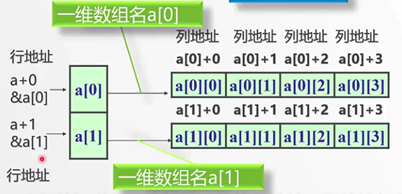问题：指针增一怎么判断是行增一还是列增一？
答：由指针类型决定！
行指针、列指针
1. 行指针：
int a;
int (*p);  // 定义一个行指针，指向一个数组，有四个元素。
p=a;   // 用行地址初始化
理解：p—>*—>—>int。先表示它是一个指针，然后让它指向一个长度为4的数组，最后说明是整型指针。因为后面括号是4，因此每次移动p++就移动4个整数，就是一行。即指针移动多少，与我们定义时指向的数组长度有关系。
区分：int *p;   // 长度为4的指针数组！与行指针不同！
规律：定义行指针一定要先把(*p)用括号括起来！后面跟的中括号表示每移动一次，移动多少个元素，即二维数组的列数是多少！（指针数组定义见后面）
问题：怎么通过行指针访问数组的每个元素？
int (*p);
p=a;
for(i=0;i<m;i++)
for(j=0;j<n;j++)
printf(“%d”,p[i][]j);   // 下标的索引方式
理解：p指向数组的首地址，因此它和数组名a完全一样，因此我们可以用指针p或数组名a访问元素。也可以用其他方式访问。但要注意的是数组名不能做自增自减操作！除此之外，指针和数组名是完全一样的！
2. 列指针：
int *p=*a;    // 用列地址初始化。
列指针就是对一个一维数组的操作，因此它和普通指针是一样的！注意：不能让p指向a，因为a是二维数组的首地址，是行地址！*a是第0行的首地址。
问题：怎么用列指针访问数组元素？
int *p=*a;   // 即p=*a; *a是第0行的首地址
for(i=0;i<m;i++)    // 行
for(j=0;j<n;j++)    // 列
printf(“%d”,*(p+i*n+j));    // 或下标索引：p[i*n+j]; 它是一个元素一个元素处理的。
总结：
(1) 二维数组首地址是行地址；
(2) 行指针指向二维数组名，行指针加减一，就移动一行；
(3) 行指针+i表示第i行的行地址，取*就转换为列地址，转为对一维数组的处理；

指针数组
int a;
int *p;
p=a;    // 错误！指针数组中没有这种初始化形式！只有行指针才能指向行地址！这里的p不是行指针！

int (*p);
p=a;    // 行指针指向二维数组行地址

int *p;    // 指针数组

指针数组：一个数组中若每个元素都是一个指针，那么称它为指针数组。
(1) 由基类型相同的指针构成；
(2) 每个元素都是一个指针；
(3) 使用前必须初始化；
(4) 常用于对多个字符串进行处理。（一维字符数组可以处理一个字符串，那么二维字符数组可以存储和处理多个字符串）
例如：
char *proname[]={“FORTRAN”,”C”,”C++”};   // 定义一个指针数组并初始化
表示：有三个字符串，因此指针数组的长度为3，即这个数组有3个元素，每个元素都是一个指针。字符串常量是存放在数据区的const存储区中，可能连续也可能不连续。proname存储了字符串常量的首地址。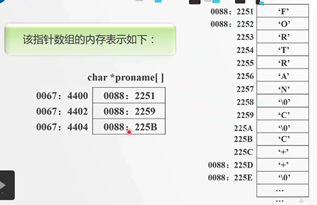在内存中的表示：
可以看出，指针数组可以处理多个字符串。指针数组的长度是多少，就可以最多处理多少个字符串。
问题：指针数组和二维字符数组有什么区别？
#define N 100   // 最多处理100个字符串
char *str[N];    // 指针数组

#define N 100   // 最多处理100个字符串
#define M 50   // 每个字符串长度不超过50
char str[N][M];    // 二维字符数组

例如：
假设有100个字符串，90个字符串的长度为2，10个字符串的长度为50。
解释：
(1) 一个是字符指针数组，每个元素都是用来存储字符串的地址。
(2) 字符串的长度可能不同，并且字符串之间可能没有关系，存储空间可能连续也可能不连续。
(3) 字符指针数组所需空间大小：100*4+90*2+10*50=1080（100个字符串的地址，每个地址占4个字节+字符串本身占据的空间）。二维字符数组所需空间：要求字符串长度最大为M，那么如果字符串长度超过M会发生越界，如果小于M，不会出错，但所需空间（1080）远小于我们产生的空间大小（5000），造成浪费。
(4) 字符指针数组元素指向的字符串可以是不规则的长度。字符二维数组的每个元素的长度必须相同，在定义时已确定。
(5) 因此可以看出，字符指针数组对空间的需求更少，更适合处理多个字符串。

展开全文• 一维数组： 假如有一维数组如下： char a; 该数组有3个元素，数据类型为char型，地址空间如下。 如果想访问数据，直接使用a、a、a取出相应地址空间的值即可 一级指针指针即地址，char *b即...c语言
• ## 指针与二维数组

千次阅读 多人点赞 2018-06-10 19:28:19
二维数组的本质 double matrix;... 令type为double，所以double matrix为typematrix，这说明，matrix是一个一维数组，有3个元素；每个元素是type，即double类型的。 m...
• 指针赋值引发的思考？ int a = { 0 }; int(*c) = &a; a 是数组，只用 a 的话，a 就代表数组首...c 是数组指针，就是指向数组指针，所以给 c 赋值 c = &a； *c自然就等于a, *c是数组a...
• 使用指针访问二维数组中的元素C语言程序设计11200C语言使用指针访问二维数组中的元素数组与指向数组的指针数组按照维度或者说按照下标的个数分为一维数组二维数组数组和变量的使用一样可以归纳为三步第一数组的定义...
• 维数组在概念上是二维的，有行和列，但在内存中所有的数组元素都是连续排列的，它们之间没有“缝隙”。以下面的二维数组 a 为例： int a = { {0, 1, 2, 3}, {4, 5, 6, 7}, {8, 9, 10, 11} }; 从概念上理解，...
• 用于比较指针数组和数组指针实现算法的差异，利用函数返回指针实现。 可作为研究C语言指针使用的范例程序。 有源代码和实现结果图。
• 一.、二维数组元素的地址 定义如下二维数组： int a = { {0,1,2,3}, {4,5,6,7}, {8,9,10,11} }； a为二维数组名，此数组有3...而它们中每个元素又是一个一维数组， 且都含有4个 元素 (相当于4列)，例如： ac语言
• 利用数组指针p将main函数中输入的二维数组a的所有元素输出， 要求先按行的顺序输出，然后再以列的顺序输出， 其中输出整型格式用 “%3d”, (测试用数据大小不要超过2位数）。 函数接口定义： void reOut(int (*p)...
• int main(){ int a={{1,2,3},{4,5,6}};... 由于p是指向指针指针，p指向个int *类型的变量，而数组名a表示数组首元素的地址&amp;a，因此p指向a。然而a并不是int *类型的变量，p应...
• 数组名代表数组的首地址，例如： int a; int *p=NULL; p=a; &amp;nbsp; &amp;nbsp; &amp;nbsp; &amp;nbsp;其中，数组名a代表数组的首地址（即&amp;amp;a），那么表达式a+i代表数组...
• 文章目录一维数组指针和二维数组指针的定义一维数组指针和二维数组指针用于索引数组元素一维数组指针和二维数组指针的可视化解读 一维数组指针和二维数组指针的定义 一维数组指针的定义就是普通指针的定义 指针变量...c语言
• 通过指针来访问一维数组，二维数组，多维数组 */ #include const int COLS=3; const int COUNT=4; //通过一级指针，计算偏移量来遍历一维数组 void printSingleDimensionalArray(int *data,int data_len); //通过一...
• 一维数组的思路处理二维数组，将二维数组名作为二级指针传入 出现异常（图示运行在DEVC++中） 二维数组名+1相当于在行上+1 然而传入的二级指针 按二维指针指针传入的num失去了二维数组的性...C语言
• //利用指针输出一维数组任意列的值 #include&lt;stdio.h&gt; int main() { int array={1,2,3,4}; int (p); p=&amp;array; int i; scanf("%d",&amp;i); printf("%d",(*§+...
• 一、多维数组元素的地址 设有一个二维数组a，它有3行4列。它的定义为： ...而每一元素又是一个一维数组，它包含4个元素(即4个列元素)，例如，a所代表的一维数组又包含4个元素： a， a...
• 、二维数组的地址不能赋给二级指针的原因 int arr[] = { { 1,2,3 },{ 4,5,6 },{ 7,8,9 } }; int** p = arr; 二、指针遍历二维数组 int* p1 = arr; for (int i = 0; i < sizeof(arr)/sizeof(arr); ...
• LOGO 数组与指针一维数组地址和指针 主讲人周芸 教学目标 掌握一维数组的指针的用法 理解一维数组指针的本质 具备应用一维数组指针编程的能力 张丽去排队打疫苗医生通过叫号的方式依次注射 引入 一指向一维数组的...
• 一、简述一维数组与指针 （1）在C语言中，数组是占用一串连续的存储单元。数组中每个元素都可以看作一个变量，因此每个数组元素都有一个地址。 那么：int *p,a={1,2,3,4,5}; 指针p的基类型是int,数组a的各个...新手c语言
• 先上个简单的代码，对比一下普通的声明数组指针声明数组 int a0; for (int i = 0; i < sizeof(a0)/sizeof(*a0); i++) { a0[i] = i; } for (int i = 0; i < sizeof(a0) / sizeof(*a0)...
• 数组与指针一维指针）的区别就是： 1、数组是一段已经分配了内存空间的指针，而指针则是尚未分配内存空间的指针，所以数组是定长的，指针是不定长的 二维： 二维数组实际上是一个（一级指针数组），其中的...
• 如下所示，这是个简单的二维数组指针访问： #include <iostream> #define X 7 using namespace std; int main() { int a[X][X];//定义数组 for(int i=0;i<X;i++) for(int j=0;j<X;j++) { a[i]...c++...